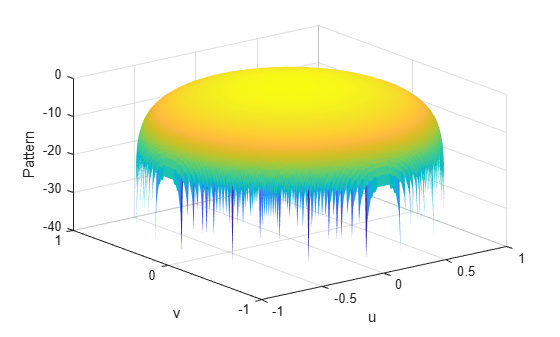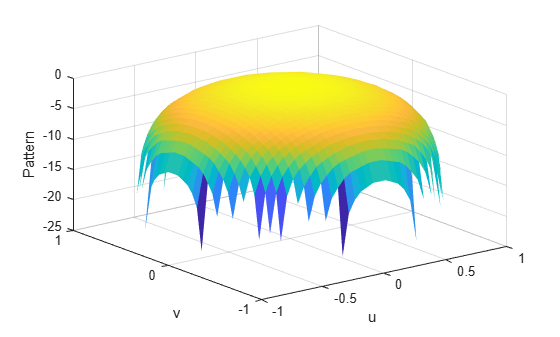Documentation

# phitheta2uvpat

Convert radiation pattern from phi/theta form to u/v form

## Syntax

``pat_uv = phitheta2uvpat(pat_phitheta,phi,theta)``
``pat_uv = phitheta2uvpat(pat_phitheta,phi,theta,u,v)``
``````[pat_uv,u_pat,v_pat] = phitheta2uvpat(___)``````

## Description

example

````pat_uv = phitheta2uvpat(pat_phitheta,phi,theta)` expresses the antenna radiation pattern `pat_phitheta` in u/v space coordinates instead of φ/θ angle coordinates. `pat_phitheta` samples the pattern at φ angles in `phi` and θ angles in `theta`. The `pat_uv` matrix uses a default grid that covers u values from –1 to 1 and v values from –1 to 1. In this grid, `pat_uv` is uniformly sampled with a step size of 0.01 for u and v. The function interpolates to estimate the response of the antenna at a given direction. Values in `pat_uv` are NaN for u and v values outside the unit circle because u and v are undefined outside the unit circle.```

example

````pat_uv = phitheta2uvpat(pat_phitheta,phi,theta,u,v)` uses vectors `u` and `v` to specify the grid at which to sample `pat_uv`. To avoid interpolation errors, `u` should cover the range [–1, 1] and `v` should cover the range [–1, 1].```

example

``````[pat_uv,u_pat,v_pat] = phitheta2uvpat(___)``` returns vectors containing the u and v coordinates at which `pat_uv` samples the pattern, using any of the input arguments in the previous syntaxes.```

## Examples

collapse all

Convert a radiation pattern to u-v form, with the u and v coordinates spaced by 0.01.

Define the pattern in terms of φ and θ.

```phi = 0:360; theta = 0:90; pat_phitheta = mag2db(repmat(cosd(theta)',1,numel(phi)));```

Convert the pattern to u-v form.

`pat_uv = phitheta2uvpat(pat_phitheta,phi,theta);`

Convert a radiation pattern to $u-v$ coordinates, with the $u$ and $v$ coordinates spaced by 0.01.

Define the pattern in terms of $\varphi$ and $\theta$.

```phi = 0:360; theta = 0:90; pat_phitheta = mag2db(repmat(cosd(theta)',1,numel(phi)));```

Convert the pattern to $u-v$ coordinates. Store the $u$ and $v$ coordinates for use in plotting.

`[pat_uv,u,v] = phitheta2uvpat(pat_phitheta,phi,theta);`

Plot the result.

```H = surf(u,v,pat_uv); H.LineStyle = 'none'; xlabel('u'); ylabel('v'); zlabel('Pattern');```Convert a radiation pattern to $u-v$ coordinates, with the $u$ and $v$ coordinates spaced by 0.05.

Define the pattern in terms of $\varphi$ and $\theta$.

```phi = 0:360; theta = 0:90; pat_phitheta = mag2db(repmat(cosd(theta)',1,numel(phi)));```

Define the set of $u$ and $v$ coordinates at which to sample the pattern. Then, convert the pattern.

```u = -1:0.05:1; v = -1:0.05:1; pat_uv = phitheta2uvpat(pat_phitheta,phi,theta,u,v);```

Plot the result.

```H = surf(u,v,pat_uv); H.LineStyle = 'none'; xlabel('u'); ylabel('v'); zlabel('Pattern');```## Input Arguments

collapse all

Antenna radiation pattern in phi/theta form, specified as a Q-by-P matrix. `pat_phitheta` samples the 3-D magnitude pattern in decibels, in terms of φ and θ angles. P is the length of the `phi` vector, and Q is the length of the `theta` vector.

Data Types: `double`

Phi angles at which `pat_phitheta` samples the pattern, specified as a vector of length P. Each φ angle is in degrees, between 0 and 180.

Data Types: `double`

Theta angles at which `pat_phitheta` samples the pattern, specified as a vector of length Q. Each θ angle is in degrees, between 0 and 90. Such angles are in the hemisphere for which u and v are defined.

Data Types: `double`

u coordinates at which `pat_uv` samples the pattern, specified as a vector of length L. Each u coordinate is between –1 and 1.

Data Types: `double`

v coordinates at which `pat_uv` samples the pattern, specified as a vector of length M. Each v coordinate is between –1 and 1.

Data Types: `double`

## Output Arguments

collapse all

Antenna radiation pattern in u/v form, returned as an M-by-L matrix. `pat_uv` samples the 3-D magnitude pattern in decibels, in terms of u and v coordinates. L is the length of the `u` vector, and M is the length of the `v` vector. Values in `pat_uv` are NaN for u and v values outside the unit circle because u and v are undefined outside the unit circle.

u coordinates at which `pat_uv` samples the pattern, returned as a vector of length L.

v coordinates at which `pat_uv` samples the pattern, returned as a vector of length M.

## More About

collapse all

### Phi Angle, Theta Angle

The φ angle is the angle from the positive y-axis toward the positive z-axis, to the vector’s orthogonal projection onto the yz plane. The φ angle is between 0 and 360 degrees. The θ angle is the angle from the x-axis toward the yz plane, to the vector itself. The θ angle is between 0 and 180 degrees.

The figure illustrates φ and θ for a vector that appears as a green solid line. The coordinate system is relative to the center of a uniform linear array, whose elements appear as blue circles.The coordinate transformations between φ/θ and az/el are described by the following equations

`$\begin{array}{l}\mathrm{sin}\left(\text{el}\right)=\mathrm{sin}\varphi \mathrm{sin}\theta \hfill \\ \mathrm{tan}\left(\text{az}\right)=\mathrm{cos}\varphi \mathrm{tan}\theta \hfill \\ \hfill \\ \mathrm{cos}\theta =\mathrm{cos}\left(\text{el}\right)\mathrm{cos}\left(\text{az}\right)\hfill \\ \mathrm{tan}\varphi =\mathrm{tan}\left(\text{el}\right)/\mathrm{sin}\left(\text{az}\right)\hfill \end{array}$`

### U/V Space

The u and v coordinates are the direction cosines of a vector with respect to the y-axis and z-axis, respectively.

The u/v coordinates for the hemisphere x ≥ 0 are derived from the phi and theta angles, as follows:

`$\begin{array}{l}u=\mathrm{sin}\theta \mathrm{cos}\varphi \\ v=\mathrm{sin}\theta \mathrm{sin}\varphi \end{array}$`

In these expressions, φ and θ are the phi and theta angles, respectively.

In terms of azimuth and elevation, the u and v coordinates are

`$\begin{array}{l}u=\mathrm{cos}el\mathrm{sin}az\\ v=\mathrm{sin}el\end{array}$`

The values of u and v satisfy the inequalities

`$\begin{array}{l}-1\le u\le 1\\ -1\le v\le 1\\ {u}^{2}+{v}^{2}\le 1\end{array}$`

Conversely, the phi and theta angles can be written in terms of u and v using

`$\begin{array}{l}\mathrm{tan}\varphi =u/v\\ \mathrm{sin}\theta =\sqrt{{u}^{2}+{v}^{2}}\end{array}$`

The azimuth and elevation angles can also be written in terms of u and v

`$\begin{array}{l}\mathrm{sin}el=v\\ \mathrm{tan}az=\frac{u}{\sqrt{1-{u}^{2}-{v}^{2}}}\end{array}$`

## See Also

### Topics

#### Hybrid Beamforming for Massive MIMO Phased Array Systems

Download the white paper